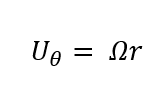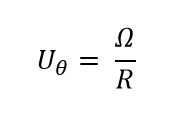top of page
Search
• Anand Zambare

# An Introduction to Vortex Dynamics

A person who is a little bit aware of fluid mechanics or who has done some basic course in fluid mechanics must have heard the word ‘Vortex’. This word is always associated with some sort of rotation of the fluid motion. It’s like whenever we think of the world cup (a cricket world cup) we think of that MS Dhoni’s six in the finish of the final. Similarly whenever we think vortex we think of rotation. But is this the story of vortex and vorticity? Or there is something very interesting waiting ahead? Let’s explore.Mathematically speaking the vorticity vector is twice the angular velocity. Now, we know that angular velocity is a first ordered tensor (Want to know more about tensors? check it here). So vorticity is also a first ordered tensor. It’s like a vector field similar to the velocity field. Now the next fundamental question is whether the velocity field is fundamental or the vorticity field is fundamental.....This question is something I would like readers to think about after going through this complete article. But I would like to make one point here, though we define "the vorticity vector as the curl of velocity vector" it is not necessary that velocity is the fundamental quantity.

While putting a point about the velocity and vorticity in the last paragraph I did write one more definition of the vorticity vector. So we can say that angular velocity is half of the curl V, where V is the velocity of the fluid domain. A vortex is commonly associated with the rotating motion of fluid around a common centerline. It is defined by the vorticity in the fluid, which measures the rate of local fluid rotation. Typically, the fluid circulates around the vortex, the speed increases as the vortex is approached and the pressure decreases. So if the angular velocity is zero then the fluid flow is said to be irrotational.

Where do we see vortices in practical situations? Vortices form in stirred fluids and may be observed in smoke rings, whirlpools in the wake of a boat, and the winds surrounding a tropical cyclone, tornado, or dust devil. The vortices do play an important role when it comes to Turbulent flows. In most vortices, the fluid flow velocity is greatest next to its axis and decreases in inverse proportion to the distance from the axis.

The story of omega i.e. angular velocity or the vorticity started with something very interesting. If we consider the incompressible flow, the equation of continuity or the mass conservation is given by div V = 0. That is the divergence of the velocity is 0. So similarly if we look at the div W or divergence of omega is zero. But here we don’t assume anything like incompressible flow or anything other. This turns out to be a purely mathematical argument.The continuity equation is more viewed as a physical principle not just as a mathematical argument. So with the continuity equation, there is associated physics. But in the case of div W = 0, there is no physics associated. It came out directly from mathematics and then we realized that there is some similarity in div W and div V. So this was the birth of this beautiful subject called “Vortex Dynamics”. In the 19th century, people like Helmholtz and Bio-Savarts started with the field theory for electromagnetic fields. Eventually, they realized the equivalence of that subject with vortex dynamics. From that point, the development in this subject started.

Let’s now look at the types of vortices. As I said people with a fundamental course in fluid mechanics must be aware of two types of vortices “free” and “forced”. So we will look at each of them separately. The governing physics for both types of vortices are different and hence to be treated completely differently. Before we look at the detailed physics of both we should understand some quantity associated with vortices and that is called “Circulation”.

Circulation in general is defined as a line integral of a vector field over a closed curve. So in fluid mechanics, if we take a line integral of velocity over a closed curve we get circulation. In electrodynamics, it is an electric charge and magnetism is a magnetic field. Since omega is the curl of V and by using stokes theorem we can convert the closed-loop line integral to area integral so by using it we convert the integral in terms of omega.Forced Vortex: This name itself is self-explanatory. The vortices are created by forces from external agencies. In such cases, we see centripetal (or centrifugal) accelerations. Here the velocity of any material point is directly proportional to the distance “r” from the center of the vortex. In such cases, we may observe the behavior of fluid elements rotating like a solid body rotation. There is more to the dynamics part of such vortices but it’s beyond the scope of this article. For forced vortex circulation taken with any closed loop will be nonzero.Free Vortex: Free vortices are also known as irrotational vortices. Yaa, you read it correctly, maybe I should wait for some time to sink it in. So going ahead into the free vortex, free vortices induce the velocity in an inverse manner with respect to “r” where r is the distance from the center of the vortex. Though the velocity is inversely proportional as we come closer to the center the magnitude increases very fast and theoretically it becomes equal to infinity. So the center of such a vortex is called a point of singularity. In the reality, the modeling of such vortices is done in a very different way. It is modeled as the forced vortex for some threshold value of r and free vortex after that value. The value of circulation for this case is zero without including the point of singularity and is nonzero when the point of singularity is included.Till this point, we have gathered so much information regarding this new topic of vortex dynamics so we will just do a recap of this article and we will look at more interesting topics in the next coming up articles.

Summary:

• ·A vortex is commonly associated with the rotating motion of fluid around a common centerline. And it measures the rate of local fluid rotation.

• Vortices form in stirred fluids and may be observed in smoke rings, whirlpools in the wake of a boat, and the winds surrounding a tropical cyclone, tornado, or dust devil.

• Similar to what we have in incompressible flows i.e. div V = 0 we have div W = 0. But the div W = 0 is a pure mathematical argument.

• Vortices are of two types Free and Forced vortices. In forced vortex velocity is directly proportional to the radius and in free it is inversely proportional to the radius.

• Circulation is defined as the closed-loop integral of the vector field. For vortex dynamics that field is velocity field.

• ·Circulation is zero for free vortex excluding the point of singularity and is non zero including the point of singularity in case of the free vortex. It is always nonzero for forced vortex.

Credits: The image of the tornado and gif used is taken from Wikipedia. All the credits to it.